Next: Example 2 Up: Examples Previous: Examples   Contents

Example 1

Consider the equation: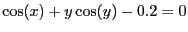which defines a curve in the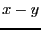plane on which you want to determine the closest point to the origin when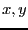lie in the range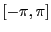. This leads to trying to find the minimum of the function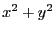under the constraint.

The procedure for the interval evaluation of the 2 functions will be written as:

INTERVAL_VECTOR IntervalTestFunction (int l1,int l2,INTERVAL_VECTOR & in)
// interval valued test function
{
INTERVAL x,y;
INTERVAL_VECTOR xx(2);

x=in(1);
y=in(2);
if(l1==1)
xx(1)=Cos(x)+y*Sqr(Cos(y))-0.2;
if(l1<=2 && l2>=2)
xx(2)=Sqr(x)+Sqr(y);
return xx;
}
while the main program may be written as:

int main()
{
int Iterations, Dimension,Dimension_Eq,Num,i,j,precision;
double Accuracy,Accuracy_Variable;
INTERVAL_MATRIX SolutionList(2,2);
INTERVAL_VECTOR TestDomain(2),F(2),P(2),H(2);
INTEGER_VECTOR Type(2);
INTERVAL Optimum;
REAL pi;

pi=Constant::Pi;

Dimension_Eq=2;Dimension=2;
TestDomain(1)=INTERVAL(-pi,pi);TestDomain(2)=INTERVAL(-pi,pi);

cerr << "Number of iteration = "; cin >> Iterations;
cerr << "Accuracy on Function = "; cin >> Accuracy;

Type(1)=0;Type(2)=-2;
Accuracy=0;

Num=Minimize_Maximize(Dimension,Dimension_Eq,Type,
IntervalTestFunction,TestDomain,Iterations,Accuracy_Variable,
Accuracy,0,Optimum,SolutionList);
if(Num<0)
{
cout << "The procedure has failed, error code:"<<Num<<endl;
return 0;
}
cout<<"Optimum:"<<Optimum<<" obtained at"<<endl;
for(i=1;i<=Num;i++)
{
cout << "x=" << SolutionList(i,1) <<endl;
cout << "y=" << SolutionList(i,2) <<endl;
}
return 0;
}
The Minimize_Maximize and Minimize_Maximize_Gradient procedures will return the same numerical results but the number of boxes will change. The results obtained for a full bisection,
the MAX_MIDDLE_FUNCTION_ORDER and according to the accuracy epsilonf and the storage mode (either direct (DSM) or reverse (RSM), see section 2.3.1.2) are presented in the following table (the number of boxes for the Minimize_Maximize_Gradient procedure is indicated in parenthesis):
 epsilonf Minimum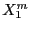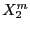boxes 0.01, DSM [1.12195667, 1.12195667] [-0.944932,-0.4786] [-0.944932,-0.4786] 76 (36) 0.01, RSM [1.12195667, 1.12195667] [-0.944932,-0.4786] [-0.944932,-0.4786] 59 (37) 0.001, DSM [1.1401661,1.1401661] [-0.954903,-0.477835] [-0.954903,-0.477835] 201 (75) 0.001, RSM [1.1401661,1.1401661] [-0.954903,-0.477835] [-0.954903,-0.477835] 148 (67) 0.000001, DSM [1.14223267,1.14223267] [-0.957596,-0.474596] [-0.957596,-0.474596] 5031 (2041) 0.000001, RSM [1.14223267,1.14223267] [-0.957596,-0.474596] [-0.957596,-0.474596] 4590 (2164)
This example shows clearly the influence of the constraint equation on the determination of the optimum.Next: Example 2 Up: Examples Previous: Examples   Contents
Jean-Pierre Merlet 2012-12-20